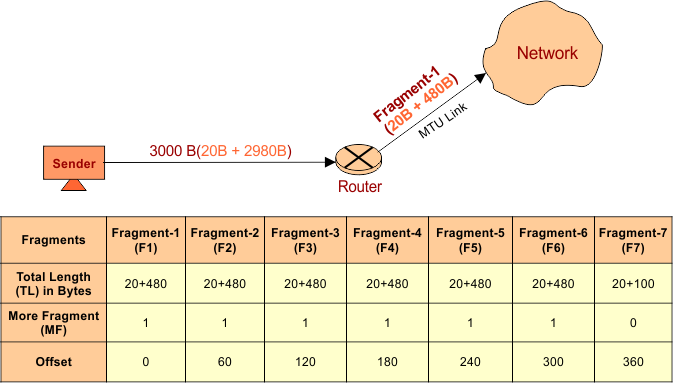Select Page

Question: A datagram of 3000 bytes (20 bytes of IP header + 2980 bytes IP payload) reached at router and must be forward to link with MTU (maximum transmission unit) of 500 bytes. How many fragments will be granted and also write MF, offset, total length value for all.

## Numerical Solution

After the fragmentation of datagram into fragments (packets), each fragment will carry the same header size which was same in original datagram. So in this question, 20 byte will be the compulsory part of each fragment.

As the limit of a link is 500 bytes to transfer in which 20byte is header size. So, 480 bytes of payload can transfer in each fragment.

### Total Number of Fragments

Total number of fragments can be calculated by considering a ceiling value of following formula

So, Total fragment = 2980 / 480 = 7

Descriptive diagram in given below### Total Length

Total length is the combination of all fragmented payloads. By adding the payload  of each fragment will always equal to the size of original payload. As

Total length of payload  = 480+480+480+480+480+480+100 = 2980.

Note: Header length will remain the same 20 bytes at receiving end. So, 20+2980 bytes = 3000 bytes. It was the original datagram of 3000 bytes.

### More Fragments (MF)

MF value may be 0 or 1.

• When MF bit value is 0 then It tells to the receiver that the current datagram-fragment is the last fragment and no more segment will appear of same datagram.
• When MF bit value is 1 then it tells more fragments are still to come after this fragment.

### Fragment Offset

It use scaling factor of 8. Fragment offset can be calculated by using the following formula

Fragment offset for a given fragmented IP datagram = Number of data (payload) bytes ahead of it / 8

• At fragment F1, no byte is already transferred. So fragment offset = 0/8 B = 0
• At fragment F2, 480 bytes of F1 is already transferred. So fragment offset = 480/8 B = 60
• At fragment F3, 480 bytes of F1 and F2 is already transferred. So fragment offset = 480*2/8 B =120
• At fragment F4, 480 bytes of F1,F2 and F3 is already transferred. So fragment offset = 480*3/8 B =180
• At fragment F5, 480 bytes of F1,F2,F3 and F4 is already transferred. So fragment offset = 480*4/8 B =240
• At fragment F6, 480 bytes of F1,F2,F3,F4 and F5 is already transferred. So fragment offset = 480*5/8 B =300
• At fragment F7, 480 bytes of F1,F2,F3,F4,F5 and F6 is already transferred. So fragment offset = 480*6/8 B =360

Help Other’s By Sharing…